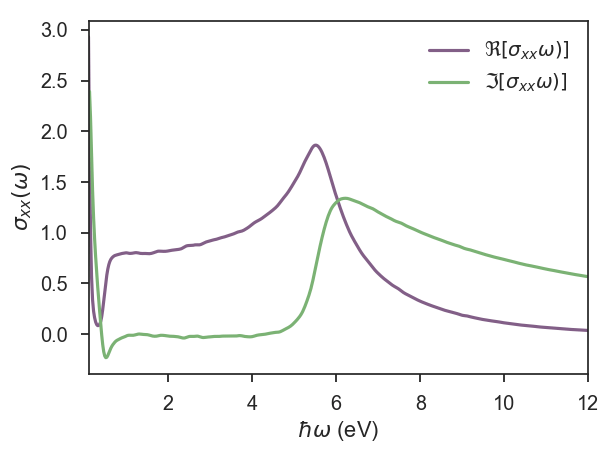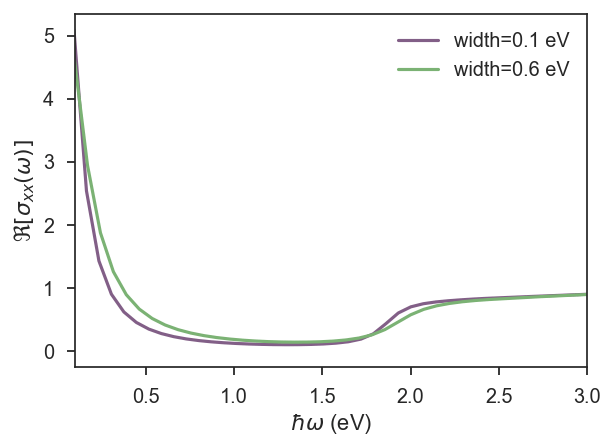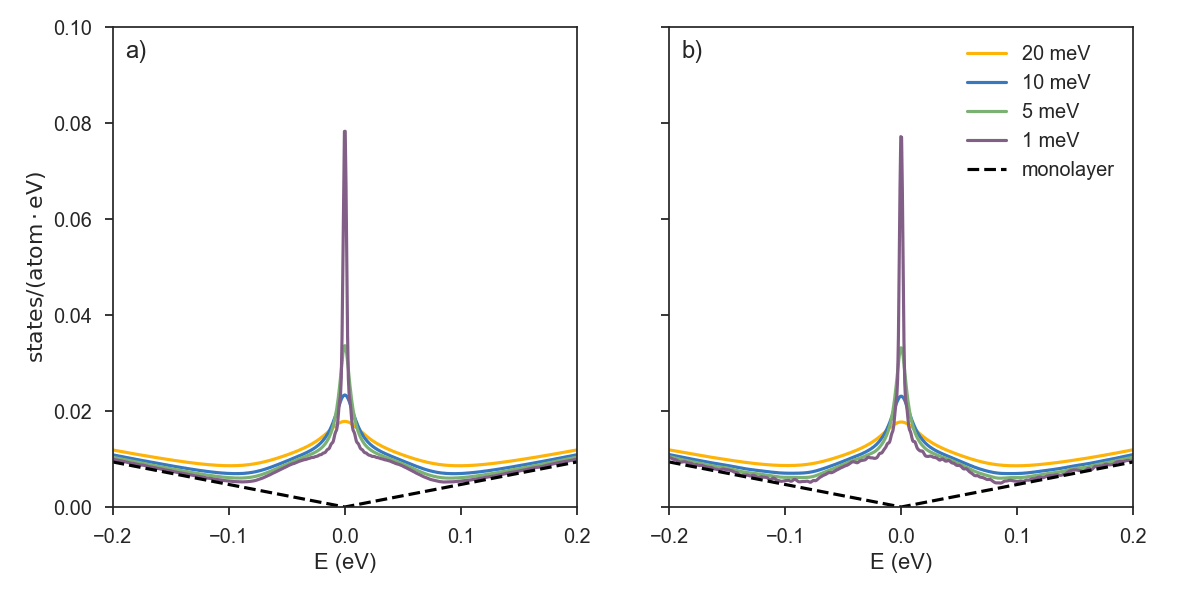# Phosphorene: DC conductivity along different directions

This is a small tutorial to illustrate the use of KITE to investigate materials with anisotropic electrical conductivity. To this end, we consider a simplified tight-binding model for single layer phosphorene 1. Even though this model is very simple, it captures the anisotropic band structure of phosphorene, which is Dirac like in one direction and Schrödinger like in the other direction. This behaviour results in highly anisotropic transport properties along the different directions 2.

Here, we calculate the single energy longitudinal conductivity (singleshot_conductivity_dc) in the vicinity of the band gap and show that a fast numerical calculation, that is set to run in a normal laptop for about 3-4 minutes, can reproduce qualitatively the expected anisotropic conductivity along xx and yy directions.

Here, we highlight parts of the python scripts. The complete scripts can be downloaded here for the xx conductivity and here for the yy conductivity .

After the imports that are necessary for KITE, we define the lattice, with Pybinding syntax:

def monolayer_4band(num_hoppings=4):
"""Monolayer phosphorene lattice using the four-band model

Parameters
----------
num_hoppings : int
Number of hopping terms to consider: from t2 to t5.
"""
a = 0.222  # nm
ax = 0.438  # nm
ay = 0.332  # nm
theta = 96.79 * (pi / 180)
phi = 103.69 * (pi / 180)

lat = pb.Lattice(a1=[ax, 0], a2=[0, ay])

h = a * sin(phi - pi / 2)
s = 0.5 * ax - a * cos(theta / 2)
('B', [ s/2,        -ay/2, 0], 0),
('C', [-s/2 + ax/2,     0, 0], 0),
('D', [ s/2 + ax/2,     0, h], 0))

lat.register_hopping_energies({'t1': -1.22, 't2': 3.665, 't3': -0.205,
't4': -0.105, 't5': -0.055})

if num_hoppings < 2:
raise RuntimeError("t1 and t2 must be included")
elif num_hoppings > 5:
raise RuntimeError("t5 is the last one")

if num_hoppings >= 2:
([-1, -1], 'A', 'D', 't1'),
([ 0,  0], 'B', 'C', 't1'),
([ 0, -1], 'B', 'C', 't1'))
lat.add_hoppings(([ 0,  0], 'A', 'B', 't2'),
([ 0,  0], 'C', 'D', 't2'))
if num_hoppings >= 3:
lat.add_hoppings(([ 0,  0], 'A', 'D', 't3'),
([ 0, -1], 'A', 'D', 't3'),
([ 1,  1], 'C', 'B', 't3'),
([ 1,  0], 'C', 'B', 't3'))
if num_hoppings >= 4:
lat.add_hoppings(([ 0,  0], 'A', 'C', 't4'),
([ 0, -1], 'A', 'C', 't4'),
([-1,  0], 'A', 'C', 't4'),
([-1, -1], 'A', 'C', 't4'),
([ 0,  0], 'B', 'D', 't4'),
([ 0, -1], 'B', 'D', 't4'),
([-1,  0], 'B', 'D', 't4'),
([-1, -1], 'B', 'D', 't4'))
if num_hoppings >= 5:
([-1,  0], 'C', 'D', 't5'))

lat.min_neighbors = 2
return lat


This model, as defined above, can be used with different number of hoppings. The user can decide the number that is used in the calculation when defining the lattice:

lattice=monolayer_4band(num_hoppings=4)


To use the large-scale “single-shot” algorithm for direct evaluation of zero-temperature DC conductivities, the resolvent operator requires a nonzero broadening (resolution) parameter eta, which is given in eV. As this type of calculation is energy dependent, it is also necessary to provide a list of desired energy points to the calculation object. In the single shot calculations, the computational time scales linearly with the energy points. For this example, that is intended to run in a normal desktop, we consider a small number of points and the energy range is set in the vicinity of the band gap.

The number of points and the list of energy points can be created when calling the calculation, as illustrated here:

calculation = kite.Calculation(configuration)
calculation.singleshot_conductivity_dc(energy=[(1.0 / 25 * i)*3.5  for i in range(25)],
num_moments=512, num_random=5, num_disorder=1,
direction='xx', eta=0.02)


Alternatively, one can define the number of points and the energy list outside calculation

npoints=25
epoints=[(1.0 / npoints * i)*3.5  for i in range(npoints)]

calculation.singleshot_conductivity_dc(epoints, num_moments=512, num_random=5,
num_disorder=1, direction='xx', eta=0.02)


Now it is time to save the configuration in a hdf file:

kite.config_system(lattice, configuration, calculation, modification, 'phxx.h5')


It is not possible to request same type of calculation in a single call. In this case, we want to calculate the conductivity in xx and yy directions where the type of the calculation is the same, which means we need another hdf file for yy conductivity.

Let’s repeat the procedure for another direction:

calculation.singleshot_conductivity_dc(epoints, num_moments=512, num_random=5,
num_disorder=1, direction='xx', eta=0.02)
kite.config_system(lattice, configuration, calculation, modification, 'phyy.h5')



For completeness, we provide the two python scripts for both orientations.

The result of this fast calculation can be seen in the figure below, for l1=512, l2=512. To get a feeling of how KITE works, we suggest modifying parameters like eta and num_random.In the next figure, we repeat the calculation for 300 energy points and 10 random vectors and a large energy window.1 Alexander N. Rudenko, Mikhail I. Katsnelson, Phys. Rev. B 89, 201408 (2014)

2 H. Liu, A. T. Neal, Z. Zhu, X. Xu , D. Tomanek and P. D. Ye, ACS Nano 8, 4033 (2014) .

# Disordered Haldane model and the transverse conductivity

Haldane Hamiltonian is a single-orbital tight-binding model on a honeycomb lattice with a sublattice-staggered on-site potential (orbital mass) and complex hoppings between next-nearest-neighbor sites that produce a staggered magnetic field configuration with vanishing total flux through the unit cell 1. This model is a Chern insulator (or a quantum anomalous Hall insulator because it hosts integer quantum Hall effect in the absence of an external magnetic field). This characteristic makes Haldane model ideal for illustrating another capability of KITE: the calculation of transverse conductivities.

Let us begin with the definition of the Hamiltonian for the case of pure imaginary next-nearest-neighbor hoppings:

def haldane():
"""Return the lattice specification for haldane model"""
a = 0.24595 #lattice constant
a_cc = a/sqrt(3)  #distance between neighbors
t = -1      #  nearest neighbour hopping
t2 = 0.1t/    #strength of the complex hopping
m=0.1 #orbital mass
# create a lattice with 2 primitive vectors
lat = pb.Lattice(
a1=[a, 0],
a2=[a/2, a/2 * sqrt(3)]
)

# name and position
('A', [0, -a_cc/2],m), #onsite potential (orbital mass)
('B', [0,  a_cc/2],-m)
)

# inside the main cell
([0,  0], 'A', 'B', t),
# between neighboring cells
([1, -1], 'A', 'B', t),
([0, -1], 'A', 'B', t),
([1, 0], 'A', 'A', t2 * 1j), #complex next-nearest hop.
([0, -1], 'A', 'A', t2 * 1j),
([-1, 1], 'A', 'A', t2 * 1j),
([1, 0], 'B', 'B', t2 * -1j),
([0, -1], 'B', 'B', t2 * -1j),
([-1, 1], 'B', 'B', t2 * -1j)
)

return lat


With the definition of our model, we can include different types of disorder, as documented here. For simplicity, we consider onsite uniform disorder distribution with width of 0.6 eV and zero average onsite energy (Anderson disorder):

disorder = kite.Disorder(lattice)


Now we are ready to calculate the Hall conductivity. After defining kite.configuration, as explained in Getting Started documentation, we can set kite.calculation. The post-processing tool uses the energy bounds from the density of state to perform the integration in energy, so it is better to couple conductivity with DOS:

calculation.dos(num_points=1000, num_moments=512, num_random=10, num_disorder=1)

calculation.conductivity_dc(num_points=1000, num_moments=256, num_random=50,
num_disorder=1, direction='xy', temperature=50)


This is a full spectral calculation where KITEx calculates the coefficients of the Chebyshev expansion and KITEtools uses that moments to calculate the transverse conductivity. Both temperature and num_points are parameters used by KITEtools and it is possible to modify them without running KITEx again. This type of calculation typically requires more RAM memory than DOS or single-shot DC conductivity, which imposes limitations to the sizes of the systems (that still can reach large scales with available memory). The relative errors of the stochastic trace evaluation (STE) scales with the inverse of the system size, which means that full spectrum conductivities typically require more random vectors to decrease the relative error of the STE. The relative error of the STE also depends on the Hamiltonian and the calculated quantities. Transverse conductivities have more fluctuations, at least in part of the spectrum outside the topological gap, and this tutorial illustrates this issue.

Fig. 1 shows the density of states, the longitudinal and transverse conductivity for a small lattice of Haldane model in a calculation that took 3 minutes on a laptop. KITEx captures the anomalous quantum Hall plateau extremely well, with a relative error of less than 0.1%. But it is also clear that the transverse conductivity presents significantly more fluctuations outside the plateau than the longitudinal conductivity, and we already considered 50 random vectors.We now focus on strategies to decrease the fluctuations. Depending on the computational resources, one possibility is increasing the system size. It is also possible to increase the number of random vectors. This is illustrated in Fig. 2.Finally, there are other physical ways of damping them: temperature and disorder. The use of these last two resources depend on the goals of the numerical calculation. In the present case, where we wanted to see the quantum anomalous Hall plateau, we can simply consider Anderson disorder and work with intermediate temperatures. To get a handle of how KITE works, we suggest the user to get the full script for this calculation and play with variations of system size, number of random vectors, disorder and temperature.

1 F. D. M. Haldane, [Phys. Rev. Lett. 61, 2015 (1988)](http://link.aps.org/doi/10.1103/PhysRevLett. 61.2015).

2 J. H. García, L. Covaci, and T. G. Rappoport, Phys. Rev. Lett. 114, 116602 (2015) (Supplementary material)

# Optical Conductivity: Graphene with Gaussian Disorder

KITE also calculates the optical conductivity of a given lattice for a given Fermi energy. To illustrate this capability, we calculate the optical conductivity of disordered graphene, that can be compared qualitativelly with previous results 1.

Instead of defining the lattice in our python script, we can use of one the pre-defined lattices from pybinding:

from pybinding.repository import graphene

lattice = graphene.monolayer()


To illustrate a different type of disorder, we random on-site energies that follow a Gaussian distribution

disorder = kite.Disorder(lattice)


where we define the type of statistical distribution, the sublattices they are located, the mean value of the distribution and its width.

After configuring the system, as presented in section getting started, it is time to set the calculation:

calculation = kite.Calculation(configuration)

calculation.conductivity_optical(num_points=1000, num_disorder=1,
num_random=20, num_moments=512, direction='xx')


The optical conductivity can also be calculated in different directions, which can be quite interesting in the case of Hamiltonians with non-trivial topology that also present transverse optical conductivity. However, in this example we focus on longitudinal optical conductivity. The other quantities that can be set in the python script are the same of the density of states: number of energy poins used in KITE-tools, moments in the expansion, random vectors and disorder realisations.

When calculating the optical conductivity, it is necessary to pass the Fermi energy as a parameter to the executable KITE-tools. Run ./KITE-tools –help for syntax examples.

The results of the real and imaginary parts of the optical conductivity presented in the first figure were obtained on a normal desktop with calculations that took 8 minutes for a system with $N=512\times 512$ units cells and 512 expansion moments.For systems sizes of $N=1536\times 1536$ unit cells and two different Gaussian widths, we show $\Re [\sigma_{xx}(\omega)]$ for low frequencies, where we can see Drude’s peak for $\omega\rightarrow 0$ and the onset of interband transitions at $\hbar \omega>2 E_F$2.The complete python script for this calculation can be found here

1 Shengjun Yuan, Rafael Roldán, Hans De Raedt, Mikhail I. Katsnelson, Phys. Rev. B 84, 195418 (2011).

2 T. Stauber, N. M. R. Peres, A. K. Geim, Phys. Rev. B 78, 085432 (2008).

# Large Systems

Up to this point, the idea of the tutorial was to show simple examples that could be reproduced on a desktop computer or a small work-station. But the purpose behind developing KITE was in building a flexible software for systems exceeding billion of atoms. Hence, in the following tutorial we will tackle the simulation of two different systems with their size being in the micrometer range.

# Graphene lattice with vacancy disorder

We simulated two graphene vacancy disordered systems, having more than 8.9 (large) and 0.5 (small) billion of atoms. The concentration of vacancy defects is 0.1%, while the number of moments used in the calculation is 15000. The two subplots show the zero energy modes in the density of state that are seen in graphene with diluted concentration of vacancies.
The system in subplot (a) has size of l1 = 65536; l2=65536, while in subplot (b), l1 = 8064; l2=8192. The dashed curve is pristine graphene and solid curves stand for different resolutions: yellow (20 meV), blue (10 meV), green (5 meV), purple (1 meV).

There is an important difference in the two subplots that is related to the lattice size. The limiting factor for observating tiny details in the expanded spectral functions is the mean level spacing given by the system size. This can be seen when simulating a small lattice with high-resolution, as in the case of subplot (b) with 1 meV resolution: oscillations in the DOS are a sign that we are getting into the limit of showing the quantized nature of a finite size system in terms of discrete energy levels. Probing a small system with high resolution can result in non-physical effects. Working with high resolutions (and consequently large systems) is specially important when dealing with localization problems.

If you would like to reproduce these results, we suggest you to check the script honeycomb_lat_vacancy.py under the examples. For the info, RAM requirements for running DOS on a small system specified above requires ~5GB and around 20 minutes on a node with 28 cores.# Moiré pattern

The second example is twisted bilayer graphene lattice in the clean limit, with the number of atoms exceeding ~0.7 billion. The model Hamiltonian  of such a system has much larger coordination number (average number of neighbors per each atomic site), and the important paramenter when estimating the running time (and the memory requirements) is the “effective” size, the product of the number of sites and the coordination number. In that sense, this system is in the mid range, between the small and the large system of the previous example.

Depending on the rotation between adjacent layers, the resulting moiré pattern will have more or less effect on the low energy electrons in graphene. For high twist angles, van Hove singularities are far from the charge neutrality point. As the angle is decreased, they “move” to lower energies and eventually merge, forming flat bands at the Dirac point. This happens at so called “magic” angles of rotation, which are of great interest recently , as they can provide an interesting playground to study correlations in graphene, with novel states of matter ranging from Mott insulators to superconductors.

Subplots a) and b) are showing the DOS for rotation angles of 2.005 (low) and 13.741 (high) degrees respectively. As in the previous case of disordered graphene, depending on the resolution, fine details around the Dirac point appear. High twist angles give rise to properties more similar to AB graphene. At low angles, the spectrum is much richer, and the linear spectrum of the two layers is fully changed due to the induced interlayer coupling. Apart from the electron-hole asymmetry, at higher energies, both densities follow the shape of the ones of a monolayer. Similar to the previous example, highier resolutions in the calculations lead to the appearance of oscillations in the density of state. Interestingly enough, as the angle is decreased, more states appear in the low energy spectrum, which reduces the mean level spacing. For the same high resolution of 1meV in subplot b) we can see the appearance of oscillations mentioned in the previous section, while the same DOS plot in subplot a) looks very smooth. When considering an arbitrary system, both spectrum and the size reflect on the level of details you are able to distinguish, while obtaining the results with physical meaning.Below, you can find a script used to configure the KITE model for twisted bilayer.
The lattice of twisted bilayer graphene (especially at low rotation angles) has a much more complex unit cell compared to simple AB stacked bilayer, where for an arbitrary twisting one does not have the access to all the neighbors using simple neighboring distance vectors. More advanced search is needed for finding the unit cell connections. The search and the hopping calculation is done separately, because of our desire not to make this script too complex. Instead, we use an already defined lattice object that is loaded with a simple command:

# define the angle
angle = 21.787 # or 13.174, 7.341, 2.005
# define the name of the pb.Lattice object
name = 'lattice_tblg_{:.3f}'.format(angle)